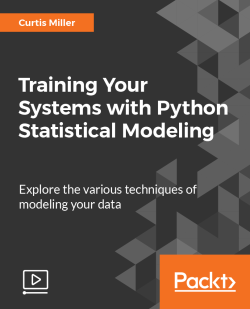### Training Your Systems with Python Statistical Modeling [Video]

By Curtis Miller
• Constantly updated with 100+ new titles each month
• Breadth and depth in over 1,000+ technologies

Python, a multi-paradigm programming language, has become the language of choice for data scientists for data analysis, visualization, and machine learning. This course takes you through the various different concepts that get you acquainted and working with the different aspects of Machine Learning.

You’ll start by diving into classical statistical analysis, where you will learn to compute descriptive statistics with Pandas. From there, you will be introduced to supervised learning, where you will explore the principles of machine learning and train different machine learning models. Next, you’ll work with binary prediction models, such as data classification using K-nearest neighbors, decision trees, and random forests.

After that, you’ll work with algorithms for regression analysis, and employ different types of regression, such as ridge and lasso regression, and spline interpolation using SciPy. Then, you’ll work on neural networks, train them, and employ regression on neural networks. You’ll be introduced to clustering, and learn to evaluate cluster model results, as well as employ different clustering types such as hierarchical and spectral clustering. Finally, you’ll learn about the dimensionality reduction concepts such as principal component analysis and low dimension representation.

# Style and Approach

This course balances in-depth content with tutorials that put the theory into practice. This course will give you both a theoretical understanding and practical examples that show you the art of statistical modeling and training with the help of Python’s various tools and packages.

Publication date:
January 2018
Publisher
Packt
Duration
4 hours 5 minutes
ISBN
9781788293402